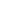Share

# The Leet Sheet

Leet or "leetspeak" is a variation of the English alphabet used primarily on the Internet. The table below contains characters and groups of characters that can be used as substitutes for letters in leetspeak. Each letter has multiple replacement options, so leet words often have several possible variations. For example, the word "leet" itself can be expressed as "l33t" or "1337."

Letter Leet Variations
A
• @
• 4
• ^
• /\
• /-\
• aye
B
• 8
• 6
• 13
• |3
• /3
• ß
• P>
• |:
C
• ¢
• <
• [
• (
• {
D
• )
• |)
• [)
• ?
• |>
• |o
E
• 3
• &
• ë
• [-
F
• ƒ
• |=
• /=
• |#
• ph
G
• 6
• 9
• &
• C-
• (_+
• gee
H
• #
• }{
• |-|
• ]-[
• [-]
• )-(
• (-)
• /-/
I
• 1
• !
• ¡
• |
• ]
• eye
J
• ]
• ¿
• _|
• _/
• </
• (/
K
• X
• |<
• |{
• |(
L
• |
• 1
• £
• |_
• 1_
• ¬
M
• |v|
• |\/|
• /\/\
• (v)
• /|\
• //.
• ^^
• em
N
• |\|
• /\/
• [\]
• <\>
• /V
• ^/
O
• 0
• ()
• []
• °
• oh
P
• |*
• |o
• |"
• |>
• 9
• |7
• |^(o)
Q
• 9
• 0_
• ()_
• (_,)
• <|
R
• 2
• ®
• /2
• 12
• I2
• l2
• |^
• |?
• lz
S
• 5
• \$
• §
• z
• es
T
• 7
• +
• -|-
• ']['
U
• µ
• |_|
• (_)
• L|
• v
V
• \/
• ^
W
• VV
• \/\/
• \\'
• '//
• \|/
• \^/
• (n)
X
• %
• *
• ><
• }{
• )(
• ecks
Y
• ¥
• J
• '/
• j
Z
• 2
• 7_
• ~/_
• >_
• %

Resources Home